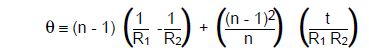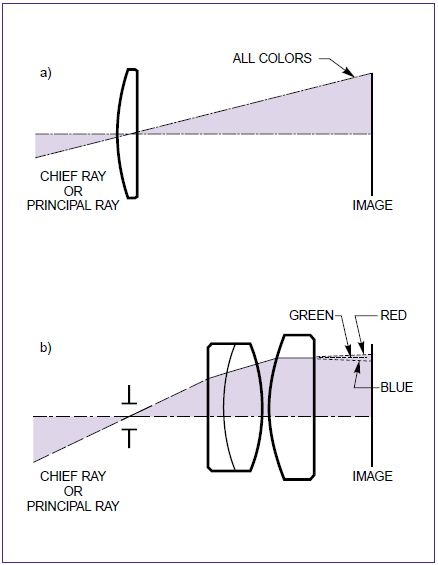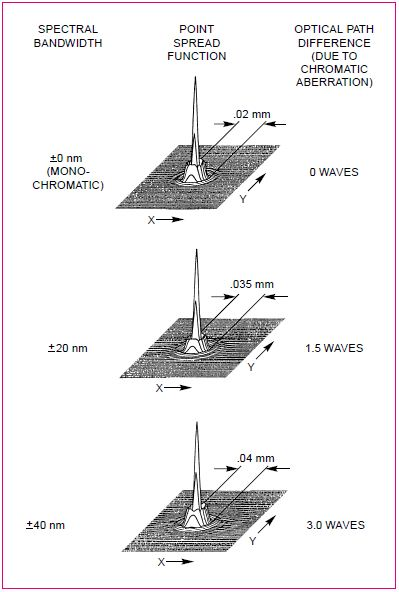# Overview of Simple Lenses

The simple lens is a single optical element that refracts incident light to form an image. The most common simple lens forms are the Plano Convex, Bi-convex, Plano Concave, and Bi-concave.

Each lens has a certain degree of power (θ):

θ = (n - 1) (1/R1 - 1/R2)

Where:
θ = Lens power (≡ 1/focal length)
n = Index of refraction of the lens material
R1 = Radius of surface 1 of lens
R2 = Radius of surface 2 of lens. For a bi-convex lens, R2 is negative.

## Example of Calculating Lens Power for Plano Convex Lens

If we had a plano convex lens made of crown glass (n = 1.52), with an R1 of 104 mm and R2 of infinity, the power would be:

θ = (1.52-1) (1/104 - 1/∞)
θ = 0.52 (0.0096)
θ = 0.005mm-1

Knowing θ, focal length is calculated as:

f = 1/ θ, f = 1/0.005 f = 200mm

## Lens Power for Bi-Convex lens

Lens power for a thin lens is given above. Thickness has no impact on the focal length of plano convex or plano concave lenses. If the lens in the example had been bi-convex, with R1 and R2 both equal to 105.8 mm, the simple formula would give a focal length of 102.4 mm for a refractive index of 1.52. Using the precise formula:Where t = Lens thickness.

The precise formula gives a focal length of 101 mm. The 1.4% difference shows that the simple formula is enough in most cases.

## Lateral Color for Simple Lenses

Lateral color is the second chromatic aberration. For on-axis light bundles, the central ray travels on the optical axis. For off-axis bundles, the central ray is called the chief ray, or principal ray. In case lateral color exists in the lens system, the principal ray is dispersed, causing the different wavelengths to image at different heights on the image plane. The result is a chromatic, radial blur for off-axis image points.Figure 1. (a) Simple lens with minimal principal ray refraction has minimal lateral color. (b) Eyepiece with substantial non-symmetrical refraction of the principal ray is afflicted with lateral color.

## Spherical Aberration and Diffraction Limited Lenses

When it is used at a single wavelength to cover a small field angle, the simple lens works. A simple lens would be fine for focusing a monochromatic collimated beam onto a detector with a 5milliradian field.

Ideally, the focused wavefront is spherical in shape. Any departure from this spherical wavefront is due to spherical aberration. The departure from the ideal is expressed in terms of waves, or fractions of a wave; one wave being equal to the wavelength of the light. The OPD, or optical path difference, is the departure from the ideal spherical wavefront.

## Lenses Used as Condensers or Low F/# Focusing Lens

While using a low F/# lens as a collimating condenser, the best source-lens distance (lens-target distance) may not be equal to the focal length. The focal length is the appropriate spacing for the paraxial rays, but the rays at the edge of the lens, the marginal rays, have a shorter focal length, the marginal focal length. This is due to spherical aberration.

Since the focal length of the simple lens depends on the refractive index, and the refractive index depends on wavelength, the lens focus varies with wavelength. This wavelength dependence severely limits the spectral bandwidth over which a simple lens can be used.

The modulation transfer function also shows the impact of chromatic aberration for the three cases, as shown in Figure 2.Figure 2. PSF of a 200mm focal length plano convex lens operating at F/8.

The MTF curves are a measure of the contrast of the image as a function of spatial frequency. A high value of the modulation at high frequency means high resolution of closely spaced image features, while the integrated value of the MTF curve is a measure of the overall image quality. Figure 3 shows the MTF curves for the three cases; monochromatic light, ±20nm and ±40nm. If it is assumed that that 0.1 modulation is adequate for resolution, then the maximum resolution for the monochromatic and ±20nm cases is 82 cycles/mm. The resolution for the ±40nm case is 70 cycles/mm. However, integration of the MTF curves results in relative areas of 1, 0.8, and 0.6. This indicates a progressively more egraded image for both the ±20nm and ±40nm cases.

Oriel Instruments, a Newport Corporation brand, was founded in 1969 and quickly gained a reputation as an innovative supplier of products for the making and measuring of light. Today, the Oriel brand represents leading instruments, such as light sources covering a broad range, from UV to IR, pulsed or continuous, and low to high power.

Oriel also offers monochromators and spectrographs, as well as flexible FT-IR spectrometers, which make it easy for users across many industries to build instruments for specific applications. Oriel is also a leader in the area of Photovoltaics with its offering of solar simulators, that allow you to simulate hours of solar radiation in minutes. Oriel continues to bring innovative products and solutions to Newport customers around the world.This information has been sourced, reviewed and adapted from materials provided by Oriel Instruments.

## Citations

• APA

Oriel Instruments. (2019, July 24). Overview of Simple Lenses. AZoOptics. Retrieved on July 03, 2020 from https://www.azooptics.com/Article.aspx?ArticleID=899.

• MLA

Oriel Instruments. "Overview of Simple Lenses". AZoOptics. 03 July 2020. <https://www.azooptics.com/Article.aspx?ArticleID=899>.

• Chicago

Oriel Instruments. "Overview of Simple Lenses". AZoOptics. https://www.azooptics.com/Article.aspx?ArticleID=899. (accessed July 03, 2020).

• Harvard

Oriel Instruments. 2019. Overview of Simple Lenses. AZoOptics, viewed 03 July 2020, https://www.azooptics.com/Article.aspx?ArticleID=899.